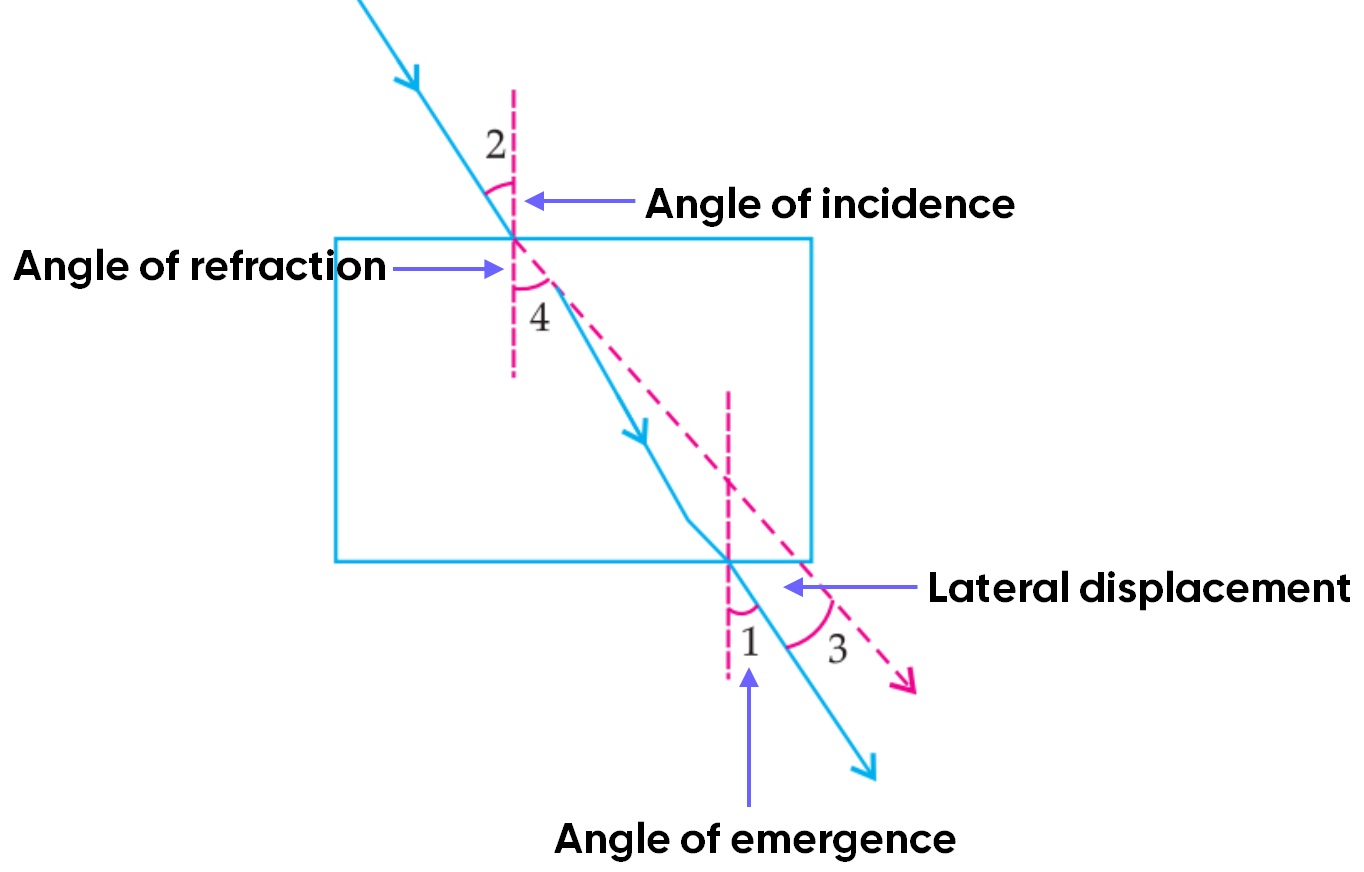MCQ from Past Year Papers

Class 10
Chapter 10 Class 10 - Light - Reflection and Refraction (Term 1)

## (d) 2, 1, 3, 4

• Angle 2 in the image is angle of incidence
• Angle 4 is the angle of refraction
• Angle 1 is the angle of emergence
• Angle 3 is the lateral displacementSo, the correct answer is (b) 2, 1, 4, 3

983 students joined Teachoo Black. What are you waiting for?